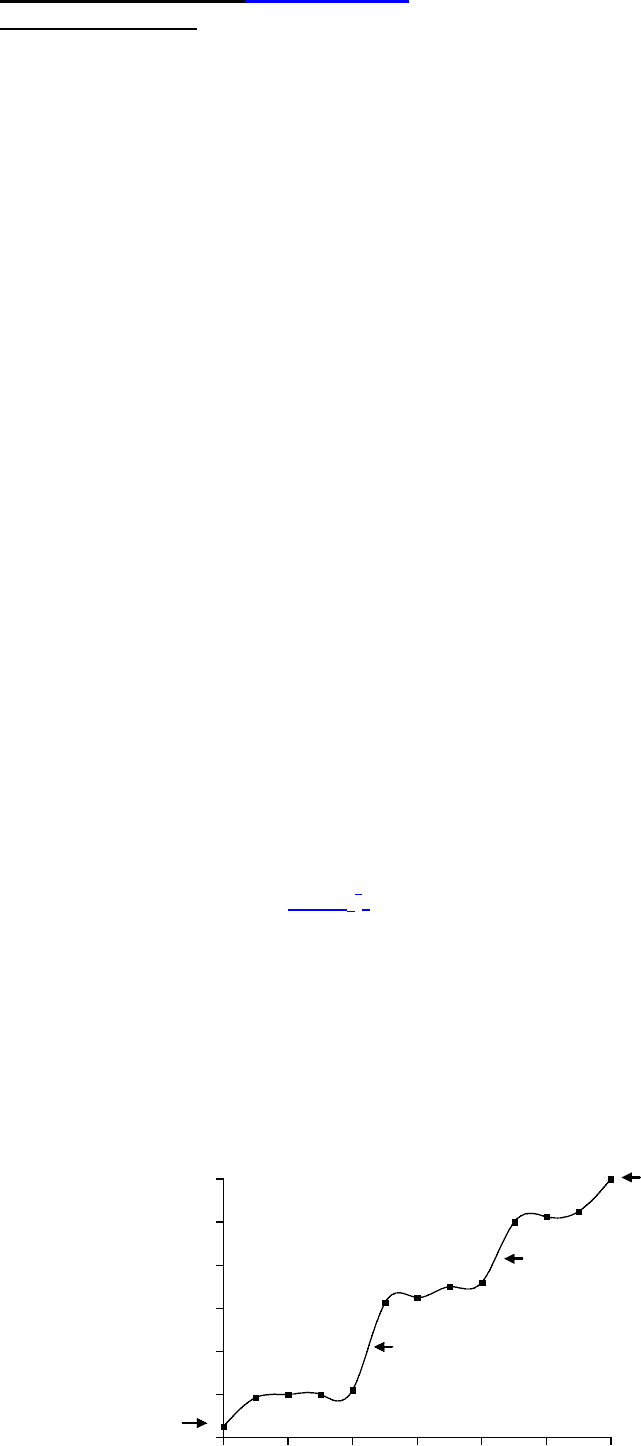Class Notes (1,100,000)
US (480,000)
UNH (600)
(5)
John (5)
Lecture 10

# BCHM 658 Lecture Notes - Lecture 10: Logarithm, Titration Curve, Sodium Hydroxide

Department
BCHM - Biochemistry
Course Code
BCHM 658
Professor
John
Lecture
10

This preview shows page 1. to view the full 4 pages of the document.Biomolecular tutorial 2 Tutors’ version
pH and buffering
1.Write down the Henderson Hasselbalch equation:
pH = pKa + log[conjugate base(A-)]/ [undissociated acid(HA)]
2. What is a buffer? Why are buffers necessary in human tissues?
Buffers are solutions which minimise pH changes when small small amounts of acid or
alkali are added, usually contain mixtures of weak acids and their salts (or weak bases
and their salts). Buffers are necessary as many metabolic processes produce acid end
products (e.g. lactic acid, CO2)which interfere with normal function of cells
3.Given that the pKa of hydrogen fluoride (HF) is 3.4, calculate the ratio of fluoride
ions (F-) to covalent HF molecules present at each of the following pH values:
i) pH 3.4 [HA] : [A-] = 1 : 1
ii) pH 5.4 [HA] : [A-] = 1 : 100
iii) pH 7.4 [HA] : [A-] = 1 : 10,000
4. The concentration of carbonic acid, H2CO3, in human plasma is about 0.00125 M.
Assuming that this is the true concentration (of the undissociated weak acid) use the
Henderson Hasselbalch equation to calculate the concentration of HCO3- in the plasma,
when the pH is 7.3.
You may assume that H2CO3 in blood has a pKa1 value of 6.1.
Using the Henderson Hasselbalch equation:
antilog (1.2) = [HCO3-] = 15.85
[H
2CO3]
Concentration of HCO3- is 15.85 x 0.00125 M = 0.02M
5 Draw a titration curve of 50 ml of 0.1M phosphoric acid with a solution of 0.1 M
NaOH
0.0 0.5 1.0 1.5 2.0 2.5 3.0
0
2
4
6
8
10
12